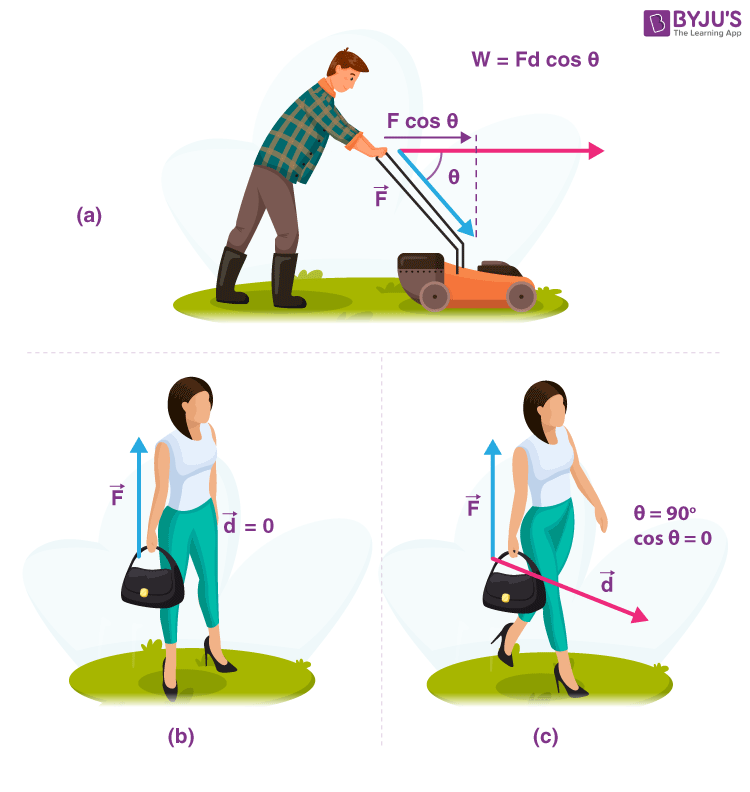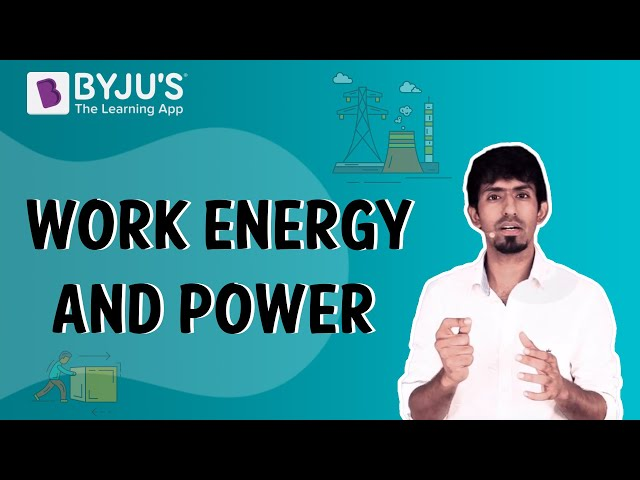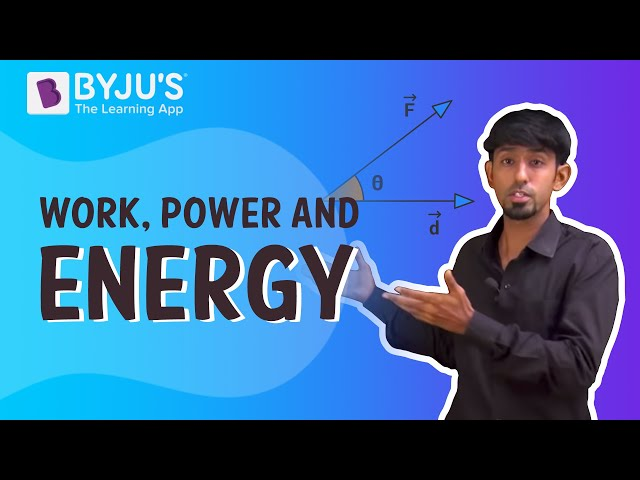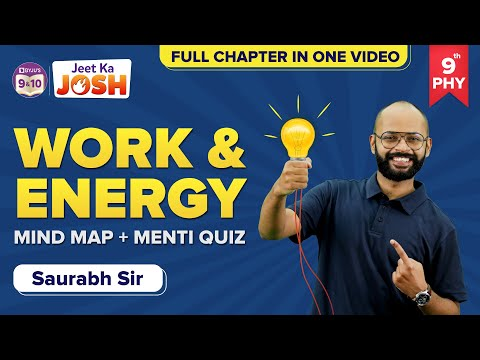# Work and Energy

Work and energy are closely related to each other. Work can also be defined as the transfer of energy. In Physics, for two objects, the work done is defined as the transfer of energy from the first object to the second object. Also, energy is defined as the capacity to do work.

Work is believed to be done by a force when an object experiences displacement parallels to the line of action of the force. It’s an activity that includes force and movement in the direction of the force. The capability for doing work is what the energy is! In this article, let’s learn more about work and energy concept along with principle of work and energy.Work is given by the equation Fdcosθ, where d is the horizontal displacement over which the force acts and θ is the angle between the force and distance.

## Principle of Work and Energy

The work-energy principle says states that

The change in kinetic energy of a body is equivalent to the net work done on the body.

This information is referred to as the work-energy principle and is derivable from the law conservation of energy.

Here K.E is the final kinetic energy and K.Ei is the initial kinetic energy.

Since you are here, you might be interested in reading the following:

## Work and Energy Equations

It is the resultant of the force applied (F) and the amount of displacement (d) and is articulated by the equation

W = F.d

Power describes the rate at which work is done. It is articulated as

P = W/t

The energy or work is articulated in Newton-meter (Nm) or Joules or kg.m2/s2. 1 Joule is defined as the amount of heat released when a force of 1 newton acts over one meter of distance.

Ever wondered what happens when the work is done against gravity?

When the work done is against gravity, the amount of work done will be equal to the product of the weight of an object and the height through which the object is lifted.

The mathematical representation of work done against the gravity is given as:

 W = m x g x h

Where,

• W is the work done
• m is the mass of an object
• g is the acceleration due to gravity
• h is the height through which the object is been lifted

## To know about work, energy and power in detail, see the videos below### Difference Between and Work Energy

Work refers to overpowering resistance by the application of force. It is evident that the resistance has been overpowered from the movement of the application of force. Energy is the capability to do work or perform some action and can be thought of as something that is possessed or stored. It exists in several forms like light, heat, electrical, potential energy, or other forms. Work and energy have similar units and are closely related. Energy can be put in storage, but that cannot be done for work.  Work is energy in motion. Energy alters from one form to another by the process of doing work.

Usually, there are two sorts of work Positive and negative work. If the direction of the force is in the same direction as the motion of its spot of application, work done is said to be positive. If the course of the force is in the opposite direction to the motion of its point of application, negative work is said to be done.

 Work Energy Work is defined as transferring energy into an object so that there is some displacement. Energy is defined as the ability to do work. Work done is always the same. Energy can be of different types such as kinetic and potential energy. The mathematical representation of work is W = F.d where F is the force applied and d is the displacement of the object. The mathematical representation of energy for kinetic energy is KE = 1/2 mv2 and for potential energy is PE = mgh where, m is the mass, v is the velocity, g is the acceleration due to gravity, and h is the height.

Hope you have understood in detail the principle of work and energy, equations, and the difference between work and energy. Stay tuned with BYJU’S for more interesting information on science and math concepts.

## The below video helps to revise the concepts in the chapter Work and Energy Class 9## Frequently Asked Questions – FAQs

Q1

### What is work?

Work is defined as transferring energy into an object so that there is some displacement.
Q2

### What is energy?

Energy is defined as the ability to do work.
Q3

### Give the mathematical representation of work done against gravity.

The mathematical representation of work done against the gravity is given as W = m x g x h
Q4

PE = mgh
Q5

### State work-energy principle?

Work-energy principle states that, the change in kinetic energy of a body is equivalent to the net work done on the body.

## The below video provides Work and Energy quizStay tuned to BYJU’S and Fall in Love with Learning!

Test your Knowledge on Work and energy